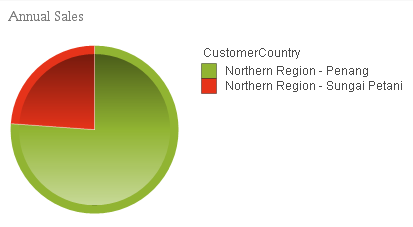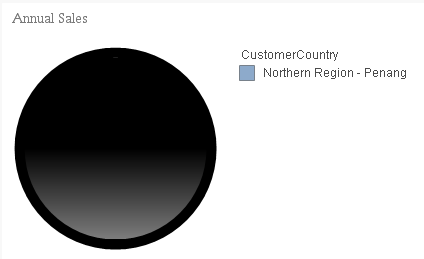QlikView Layout & Visualizations

Discussion Board for collaboration on QlikView Layout & Visualizations.

Announcements
QlikWorld 2020: Join us May 11 - 14, 2020 in Phoenix, AZ. Register early and save \$400. Learn More
HighlightedNot applicable

help on ColorMix1 function

Hi All,

In my dashboard to maintain same color combination i have used ColorMix1 function as a background expression on almost all the charts. The results are as per expectations but when selecting a single selection on any chart the color is turning to Black.

1. I am using Red and Green as my primary colors and this is working fine when there are two customer countries.2. When i click on 'Northern Region - Penang' Customer country the color on pie chart becoming Black and on legend it is blue. But i want it to be either green or red based on the sales amount.Formula i used on my expression tab is 'Sum(Sales)'

and background expression is

'ColorMix1 ((1+Sign(2*(sum(Sales)-RangeMin (top(total sum(Sales),1,NoOfRows(total))))/(RangeMax (top(total sum(Sales),1,NoOfRows(total)))-RangeMin (top(total sum(Sales),1,NoOfRows(total))))-1)*Sqrt(Fabs((2*(sum(Sales)-RangeMin (top(total sum(Sales),1,NoOfRows(total))))/(RangeMax (top(total sum(Sales),1,NoOfRows(total)))-RangeMin (top(total sum(Sales),1,NoOfRows(total))))-1))))/2, cBad,cGood)'

cBad value is =rgb(230,51,26)

CGood value is =rgb(145,180,50)

Please suggest me to resolve this issue. Any help will be more appreciated.

Regards,

Harika

Tags (4)
1 Solution

Accepted SolutionsNot applicable

Re: help on ColorMix1 function

Thank you for the explanation Gysbert, Now i understand why it is getting failed.

I have added an If condition to avoid black color and I have given a blue color which is more meaningful as the color between Legend and graph matched perfectly.

if(isnull(ColorMix1 ((1+Sign(2*(sum(Sales)-RangeMin (top(total sum(Sales),1,NoOfRows(total))))/(RangeMax (top(total sum(Sales),1,NoOfRows(total)))-RangeMin (top(total sum(Sales),1,NoOfRows(total))))-1)*Sqrt(Fabs((2*(sum(Sales)-RangeMin (top(total sum(Sales),1,NoOfRows(total))))/(RangeMax (top(total sum(Sales),1,NoOfRows(total)))-RangeMin (top(total sum(Sales),1,NoOfRows(total))))-1))))/2, cBad,cGood)),

RGB(141,170,203),

ColorMix1 ((1+Sign(2*(sum(Sales)-RangeMin (top(total sum(Sales),1,NoOfRows(total))))/(RangeMax (top(total sum(Sales),1,NoOfRows(total)))-RangeMin (top(total sum(Sales),1,NoOfRows(total))))-1)*Sqrt(Fabs((2*(sum(Sales)-RangeMin (top(total sum(Sales),1,NoOfRows(total))))/(RangeMax (top(total sum(Sales),1,NoOfRows(total)))-RangeMin (top(total sum(Sales),1,NoOfRows(total))))-1))))/2, cBad,cGood))

Regards,

Harika

4 RepliesMVP & Luminary

Re: help on ColorMix1 function

Try this:

ColorMix1 ((1+Sign(2*(sum({<CustomerCountry=>}Sales)-RangeMin (top(total sum({<CustomerCountry=>}Sales),1,NoOfRows(total))))/(RangeMax (top(total sum({<CustomerCountry=>}Sales),1,NoOfRows(total)))-RangeMin (top(total sum({<CustomerCountry=>}Sales),1,NoOfRows(total))))-1)*Sqrt(Fabs((2*(sum({<CustomerCountry=>}Sales)-RangeMin (top(total sum({<CustomerCountry=>}Sales),1,NoOfRows(total))))/(RangeMax (top(total sum({<CustomerCountry=>}Sales),1,NoOfRows(total)))-RangeMin (top(total sum({<CustomerCountry=>}Sales),1,NoOfRows(total))))-1))))/2, cBad,cGood)

talk is cheap, supply exceeds demandNot applicable

Re: help on ColorMix1 function

Hi Gysbert,

Thank you for your quick response. After i use your formula the chart stopped responding and even I am unable to go further to other customer countries.

To be more precise I am attaching sample qvw with both the pie charts on place.

When ever i try to select single Customer or Customer Country the color is black.

Regards,

HarikaMVP & Luminary

Re: help on ColorMix1 function

You're using dimension limits to show only the first five and group the rest as Other. I don't have a solution to handle such a use case. Once you select one value the colormix function won't work anymore because there's only one value of sum(Sales) left to calculate with.

talk is cheap, supply exceeds demandNot applicable

Re: help on ColorMix1 function

Thank you for the explanation Gysbert, Now i understand why it is getting failed.

I have added an If condition to avoid black color and I have given a blue color which is more meaningful as the color between Legend and graph matched perfectly.

if(isnull(ColorMix1 ((1+Sign(2*(sum(Sales)-RangeMin (top(total sum(Sales),1,NoOfRows(total))))/(RangeMax (top(total sum(Sales),1,NoOfRows(total)))-RangeMin (top(total sum(Sales),1,NoOfRows(total))))-1)*Sqrt(Fabs((2*(sum(Sales)-RangeMin (top(total sum(Sales),1,NoOfRows(total))))/(RangeMax (top(total sum(Sales),1,NoOfRows(total)))-RangeMin (top(total sum(Sales),1,NoOfRows(total))))-1))))/2, cBad,cGood)),

RGB(141,170,203),

ColorMix1 ((1+Sign(2*(sum(Sales)-RangeMin (top(total sum(Sales),1,NoOfRows(total))))/(RangeMax (top(total sum(Sales),1,NoOfRows(total)))-RangeMin (top(total sum(Sales),1,NoOfRows(total))))-1)*Sqrt(Fabs((2*(sum(Sales)-RangeMin (top(total sum(Sales),1,NoOfRows(total))))/(RangeMax (top(total sum(Sales),1,NoOfRows(total)))-RangeMin (top(total sum(Sales),1,NoOfRows(total))))-1))))/2, cBad,cGood))

Regards,

Harika